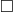# Matchings without Hall’s theorem

In practice matchings are found not by following the proof of Hall’s theorem but by starting with some matching and improving it by finding augmenting paths.  Given a matching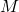in a bipartite graph on vertex classes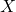and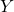, an augmenting path is a path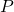from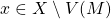to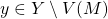such that ever other edge ofis an edge of.  Replace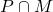by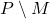produces a matching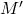with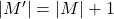.

Theorem.  Let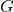be a spanning subgraph of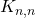.  If (i)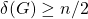or (ii)is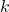-regular, thenhas a perfect matching.

Proof. Let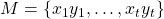be a maximal matching inwith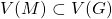.

(i) Choose,.  We have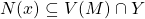and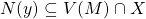.  Sincethere is ansuch that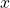is adjacent to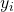and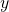is adjacent to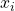.  Then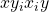is an augmenting path.

(ii) Without loss of generality,is connected.  Form the directed graph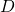on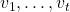by taking the directed edge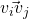(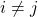) whenever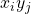is an edge of.  Add directed edges arbitrarily toto obtain a-regular digraph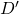, which might contain multiple edges; sinceis connected we have to add at least one directed edge.  The edge set ofdecomposes into directed cycles.  Choose a cycle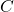containing at least one new edge of, and letbe a maximal sub-path ofcontaining only edges of.  Let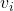,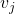be the start- and endpoints ofrespectively.  Then we can choose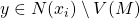and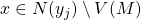, whence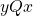is an augmenting path, where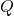is the result of “pulling back”fromto, replacing each visit to a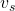inby use of the edge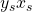of.